Journal DetailsFormat
Journal
First Published
01 Jan 2016
Publication timeframe
2 times per year
Languages
English
Copyright
© 2020 Sciendo

# Numerical Solution of Abel′s Integral Equations using Hermite Wavelet

###### Accepted: 08 May 2019
Journal DetailsFormat
Journal
First Published
01 Jan 2016
Publication timeframe
2 times per year
Languages
English
Copyright
© 2020 Sciendo

A numerical method is developed for solving the Abel′s integral equations is presented. The method is based upon Hermite wavelet approximations. Hermite wavelet method is then utilized to reduce the Abel′s integral equations into the solution of algebraic equations. Illustrative examples are included to demonstrate the validity, efficiency and applicability of the proposed technique. Algorithm provides high accuracy and compared with other existing methods.

#### MSC 2010

Introduction

Wavelets theory is a new emerging tool in applied mathematical research area. It is applicable in various fields, such as, signal analysis for waveform representation and segmentations, time-frequency analysis and Harmonic analysis. Wavelets permit the accurate representation of a variety of functions and operators. Moreover, wavelets establish a connection with fast numerical algorithms [1, 2]. Since 1991 the various types of wavelet methods have been applied for the numerical solution of different kinds of integral equations . Namely, the Haar wavelets method , Legendre wavelets method , Rationalized haar wavelet , Hermite cubic splines , Coifman wavelet scaling functions , CAS wavelets , Bernoulli wavelets , wavelet preconditioned techniques [25, 26, 27, 28,]. Some of the papers are found for solving Abel′s integral equations using the wavelet based methods, such as Legendre wavelets  and Chebyshev wavelets .

Abel′s integral equations have applications in various fields of science and engineering. Such as microscopy, seismology, semiconductors, scattering theory, heat conduction, metallurgy, fluid flow, chemical reactions, plasma diagnostics, X-ray radiography, physical electronics, nuclear physics [12, 13, 14].

In 1823, Abel, when generalizing the tautochrone problem derived the equation:

$∫0xy(t)(x−t)dt=f(x),$$$\begin{array}{} \displaystyle \int_{0}^{x} \dfrac{y(t)}{\sqrt{(x-t)}} dt=f(x), \end{array}$$

where f(x) is a known function and y(x) is an unknown function to be determined. This equation is a particular case of a linear Volterra integral equation of the first kind. For solving eq. (1) different numerical based methods have been developed over the past few years, such as product integration methods , collocation method [16, 17], homotopy analysis transform method . The generalized Abel′s integral equations on a finite segment appeared for the first time in the paper of Zeilon . There are several numerical methods for approximating the solution of singular integral equations is known. Baker  studied the numerical treatment of integral equations. A numerical solution of weakly singular volterra integral equations was introduced in . Babolian and Salimi  discussed an operational matrix method based on block-pulse functions for singular integral equations. In this paper, we introduced the Hermite wavelets based numerical method for solving Abel′s integral equations.

The article is organized as follows: In Section 2, formulation of Hermite wavelets and function approximation is presented. Section 3 is devoted the method of solution. In section 4, numerical results are demonstrated the accuracy of the proposed method by some of the illustrative examples. Lastly, the conclusion is given in section 5.

Properties of Hermite Wavelets

Wavelets constitute a family of functions constructed from dilation and translation of a single function called mother wavelet. When the dilation parameter a and translation parameter b varies continuously, we have the following family of continuous wavelets:

$ψa,b(x)=|a|−1/2ψ(x−ba),∀a,bϵR,a≠0.$$$\begin{array}{} \displaystyle \psi_{a,b} (x)=\vert a \vert^{-1/2} \psi(\dfrac{x-b}{a}),\forall a,b \epsilon R,a\neq 0. \end{array}$$

If we restrict the parameters a and b to discrete values as $a=a0−k,b=nb0a0−k,a0>1,b0>0.$$\begin{array}{} \displaystyle a=a_{0}^{-k},b=nb_{0}a_{0}^{-k},a_{0} \gt 1,b_{0} \gt 0. \end{array}$ We have the following family of discrete wavelets

$ψk,n(x)=|a|1/2ψ(a0kx−nb0),∀a,bϵR,a≠0,$$$\begin{array}{} \displaystyle \psi_{k,n}(x)=\vert a \vert^{1/2} \psi(a_{0}^{k}x-nb_{0}),\forall a,b \epsilon R, a \neq 0, \end{array}$$

where ψk,n form a wavelet basis for L2(R). In particular, when a0 = 2 and b0 = 1, then ψk,n(x) forms an orthonormal basis. Hermite wavelets are defined as 

$ψk,n(x)=2k+1/2πHm(2kx−2n+1),n−12k−1≤x$$\begin{array}{} \displaystyle \psi_{k,n}(x)= \left\{ \begin{array}{ll} \dfrac{2^{k+1/2}}{\sqrt{\pi}} H_{m}(2^kx-2n+1), & \dfrac{n-1}{2^{k-1}}\leq x \lt \dfrac{n}{2^{k-1}}\\ 0, & otherwise \\ \end{array} \right. \end{array}$$

where m = 0, 1, …, M – 1. Here is Hermite polynomials of degree m with respect to weight function W(x) = $1−x2$$\begin{array}{} \displaystyle \sqrt{1-x^2} \end{array}$ on the real line R and satisfies the following recurrence formula H0(x) = 1, H1(x) = 2x,

$Hm+2(x)=2xHm+1(x)−2(m+1)Hm(x)$$$\begin{array}{} \displaystyle H_{m+2}(x)=2xH_{m+1}(x)-2(m+1)H_{m}(x) \end{array}$$

where m = 0, 1, 2, …

Function approximation: Here we approximating the solution y(x) of Abel’s integral equations using Hermite wavelet basis as follows:

$y(x)=∑n=1∞∑m=0∞cn,mψn,m(x)$$$\begin{array}{} \displaystyle y(x)=\sum_{n=1}^{\infty} \sum_{m=0}^{\infty} c_{n,m} \psi_{n,m}(x) \end{array}$$

where ψn,m(x) is given in eq.(2). We approximate y(x) by truncating the series represented in eq.(4) as,

$y(x)=∑n=12k−1∑m=0M−1cn,mψn,m(x)=CTΨ(x)$$$\begin{array}{} \displaystyle y(x)=\sum_{n=1}^{2^{k-1}} \sum_{m=0}^{M-1} c_{n,m} \psi_{n,m}(x)=C^T\Psi(x) \end{array}$$

where C and Ψ(x) are 2k–1M × 1 matrix,

$CT=[c1,0,...c1,M−1,c2,0,...c2,M−1,...c2k−1,0,...c2k−1,M−1]$$$\begin{array}{} \displaystyle C^T=[c_{1,0},...c_{1,M-1},c_{2,0},...c_{2,M-1},...c_{2^{k-1},0},...c_{2^{k-1},M-1}] \end{array}$$

$Ψ(x)=[ψ1,0,...ψ1,M−1,ψ2,0,...ψ2,M−1,...ψ2k−1,0,...ψ2k−1,M−1]$$$\begin{array}{} \displaystyle \Psi(x)=[\psi_{1,0},...\psi_{1,M-1},\psi_{2,0},...\psi_{2,M-1},...\psi_{2^{k-1},0},...\psi_{2^{k-1},M-1}] \end{array}$$

Convergence and Error Analysis
Theorem 3.1

The series solution of Hermite wavelet $y(x)=∑n=1∞∑m=0∞cn,mψn,m(x)$$\begin{array}{} \displaystyle y(x)= \displaystyle{\sum _{n=1}^{\infty }} \sum _{m=0}^{\infty }c_{n,m} \psi_{n,m} (x) \end{array}$ is converges to y(x).

Proof

Let L2(R) be the infinite dimensional Hilbert space and ψn,m is defined as eq.(2) forms an orthonormal basis.

Let $y(x)=∑i=0M−1cn,iψn,i(x)$$\begin{array}{} \displaystyle y(x)=\displaystyle{\sum _{i=0}^{M-1}}c_{n,i} \psi_{n,i} (x) \end{array}$ where cn,i = 〈y(x), ψn,i(x)〉 for a fixed n.

Let us denote the sequence of partial sums Sn of {cn,iψn,i(x)},

Let Sn and Sm be the partial sums with nm. Now we have to prove Sn is a Cauchy sequence in Hilbert space L2(R).

Choose, $Sn=∑i=0ncn,iψn,i(x),$$\begin{array}{} \displaystyle S_{n} =\displaystyle{\sum _{i=0}^{n}}c_{n,i} \psi_{n,i} (x) , \end{array}$ Now $y(x),Sn=y(x),∑i=0ncn,iψn,i(x)=∑i=m+1ncn,i2$$\begin{array}{} \displaystyle \left\langle y(x),S_{n} \right\rangle =\left\langle y(x),\displaystyle{\sum _{i=0}^{n}}c_{n,i} \psi_{n,i} (x) \right\rangle =\displaystyle{\sum _{i=m+1}^{n}}\left|c_{n,i} \right|^{2} \end{array}$

We claim that $Sn−Sm2=∑i=m+1ncn,i2,∀n>m$$\begin{array}{} \displaystyle \left\| S_{n} -S_{m} \right\| ^{2} =\displaystyle{\sum _{i=m+1}^{n}}\left|c_{n,i} \right|^{2} ,\, \, \, \, \forall n \gt m \end{array}$

Now $∑i=m+1ncn,iψn,i(x)2=∑i=m+1ncn,iψn,i(x),∑i=m+1ncn,iψn,i(x)=∑i=m+1ncn,i2,∀n>m$$\begin{array}{} \displaystyle \left\| \displaystyle{\sum _{i=m+1}^{n}}c_{n,i} \psi_{n,i} (x) \right\| ^{2} =\left\langle \displaystyle{\sum _{i=m+1}^{n}}c_{n,i} \psi_{n,i} (x) ,\displaystyle{\sum _{i=m+1}^{n}}c_{n,i} \psi_{n,i} (x) \right\rangle =\displaystyle{\sum _{i=m+1}^{n}}\left|c_{n,i} \right|^{2} , \forall \,\, n \gt m \end{array}$

thus, $∑i=m+1ncn,iψn,i(x)2=∑i=m+1ncn,i2,∀n>m$$\begin{array}{} \displaystyle \left\| \displaystyle{\sum _{i=m+1}^{n}}c_{n,i} \psi_{n,i} (x) \right\| ^{2} =\displaystyle{\sum _{i=m+1}^{n}}\left|c_{n,i} \right|^{2} ,\, \, \, \, \forall n \gt m \end{array}$

Since, Bessel’s inequality, we have $∑i=m+1ncn,i2≤y(x)2$$\begin{array}{} \displaystyle \displaystyle{\sum _{i=m+1}^{n}}\left|c_{n,i} \right|^{2} \le \left\| y(x)\right\| ^{2} \end{array}$ is bounded and convergent.

Hence, $∑i=m+1ncn,iψn,i(x)2→0$$\begin{array}{} \displaystyle \left\| \displaystyle{\sum _{i=m+1}^{n}}c_{n,i} \psi_{n,i} (x) \right\| ^{2} \to 0 \end{array}$as m, n → ∞.

This implies, $∑i=m+1ncn,iψn,i(x)→0.$$\begin{array}{} \displaystyle \left\| \displaystyle{\sum _{i=m+1}^{n}}c_{n,i} \psi_{n,i} (x) \right\| \to 0. \end{array}$ and

Therefore {Sp} is a Cauchy sequence and it converges to s (say).

We assert that y(x) = s

Now 〈sy(x), ψn,i(x)〉 = 〈s, ψn,i(x)〉 – 〈y(x), ψn,i(x)〉 = 〈s, ψn,i(x)〉 – $limn→∞⁡Sn,ψn,i(x)=0,$$\begin{array}{} \displaystyle \left\langle \mathop{\lim }\limits_{n\to \infty } S_{n} ,\psi_{n,i} (x)\right\rangle =0, \end{array}$

This implies,

$s−y(x),ψn,i(x)=0$$$\begin{array}{} \displaystyle \left\langle s-y(x),\psi_{n,i} (x)\right\rangle =0 \end{array}$$

Hence y(x) = s and $∑i=0ncn,iψn,i(x)$$\begin{array}{} \displaystyle \displaystyle{\sum _{i=0}^{n}}c_{n,i} \psi_{n,i} (x) \end{array}$ converges to y(x) as n → ∞ and proved.

Theorem 3.2

Suppose that y(x) ∈ Cm[0, 1] and CTΨ(x) is the approximate solution using Hermite wavelet. Then the error bound would be given by,

$E(x)≤2m!4m2m(k−1)maxx∈[0,1]ym(x).$$$\begin{array}{} \displaystyle \left\| E(x)\right\| \le \left\| \frac{2}{m!4^{m} 2^{m(k-1)} } \mathop{\max }\limits_{x\in [0,1]} \left|y^{m} (x)\right|\right\| . \end{array}$$

Proof

Applying the definition of norm in the inner product space, we have,

$E(x)2=∫01y(x)−CTΨ(x)2dx.$$$\begin{array}{} \displaystyle \left\| E(x)\right\| ^{2} =\int _{0}^{1}\left[y(x)-C^{T} \Psi (x)\right]^{2} dx. \end{array}$$

Divide interval [0, 1] into 2k–1 subintervals $In=n−12k−1,n2k−1,n=1,2,3,...,2k−1.$$\begin{array}{} \displaystyle I_{n} =\left[\frac{n-1}{2^{k-1} } ,\frac{n}{2^{k-1} } \right],\, n=1,2,3,...,2^{k-1} . \end{array}$

$E(x)2=∑n=12k−1∫n−12k−1n2k−1y(x)−CTΨ(x)2dx.E(x)2≤∑n=12k−1∫n−12k−1n2k−1y(x)−Pm(x)2dx.$$$\begin{array}{} \displaystyle \left\| E(x)\right\| ^{2} =\displaystyle{\sum _{n=1}^{2^{k-1}} }\int _{\frac{n-1}{2^{k-1} } }^{\frac{n}{2^{k-1} } }\left[y(x)-C^{T} \Psi (x)\right]^{2} dx. \\\displaystyle \left\| E(x)\right\| ^{2} \le \displaystyle{\sum _{n=1}^{2^{k-1}} }\int _{\frac{n-1}{2^{k-1} } }^{\frac{n}{2^{k-1} } }\left[y(x)-P_{m} (x)\right]^{2} dx. \end{array}$$

where Pm(x) is the interpolating polynomial of degree m which approximates y(x) on In. By using the maximum error estimate for the polynomial on In, then

$E(x)2≤∑n=12k−1∫n−12k−1n2k−12m!4m2m(k−1)maxx∈Inym(x)2dx.E(x)2≤∑n=12k−1∫n−12k−1n2k−12m!4m2m(k−1)maxx∈[0,1]ym(x)2dx.E(x)2=∫012m!4m2m(k−1)maxx∈[0,1]ym(x)2dxE(x)≤2m!4m2m(k−1)maxx∈[0,1]ym(x).$$$\begin{array}{c} \displaystyle \left\| E(x)\right\| ^{2} \le \displaystyle{\sum _{n=1}^{2^{k-1} }}\int _{\frac{n-1}{2^{k-1} } }^{\frac{n}{2^{k-1} } }\left[\frac{2}{m!4^{m} 2^{m(k-1)} } \mathop{\max }\limits_{x\in I_{n} } \left|y^{m} (x)\right|\right]^{2} dx. \\\displaystyle \left\| E(x)\right\| ^{2} \le \displaystyle{\sum _{n=1}^{2^{k-1}} }\int _{\frac{n-1}{2^{k-1} } }^{\frac{n}{2^{k-1} } }\left[\frac{2}{m!4^{m} 2^{m(k-1)} } \mathop{\max }\limits_{x\in [0,1]} \left|y^{m} (x)\right|\right]^{2} dx. \\\displaystyle \left\| E(x)\right\| ^{2} =\int _{0}^{1}\left[\frac{2}{m!4^{m} 2^{m(k-1)} } \mathop{\max }\limits_{x\in [0,1]} \left|y^{m} (x)\right|\right]^{2} dx \\\displaystyle \left\| E(x)\right\| \le \left\| \frac{2}{m!4^{m} 2^{m(k-1)} } \mathop{\max }\limits_{x\in [0,1]} \left|y^{m} (x)\right|\right\| . \end{array}$$

which, we have used the well-known maximum error bound for the interpolation.

Method of Solution

Consider the Abel′s integral equation of the form,

$λy(x)=f(x)+∫0xy(t)x−tdt,0≤xt≤1$$$\begin{array}{} \displaystyle \lambda y(x)=f(x)+\int _{0}^{x}\frac{y(t)}{\sqrt{x-t} } dt,\, \, \, \, \, 0\le x\, \, t \le 1 \end{array}$$

where λ = 0 or λ = 1. We first approximate y(x) as truncated series defined in eq.(4). That is,

$y(x)≈∑n=12k−1∑m=0M−1cn,mψn,m(x)=CTΨ(x)$$$\begin{array}{} \displaystyle y(x)\approx\displaystyle{\sum_{n=1}^{2^{k-1}}} \displaystyle{\sum_{m=0}^{M-1}} c_{n,m} \psi_{n,m}(x)=C^T\Psi(x) \end{array}$$

where C and Ψ(x) are defined in eq.(6) and (7). Then substituting eq.(9) in eq.(8), we get

$λCTΨ(x)=f(x)+∫0xCTΨ(t)x−tdt$$$\begin{array}{} \displaystyle \lambda C^{T} \Psi(x)=f(x)+\int _{0}^{x}\frac{C^{T} \Psi (t)}{\sqrt{x-t} } dt \end{array}$$

Next, assume eq.(10) is precise at following collocation points $xi=2i−12kM,i=1,2,...,2k−1M.$$\begin{array}{} \displaystyle x_{i}=\dfrac{2i-1}{2^kM},i=1,2,...,2^{k-1}M. \end{array}$ Then we obtain

$λCTΨ(xi)=f(xi)+∫0xiCTΨ(t)xi−tdt$$$\begin{array}{} \displaystyle \lambda C^{T} \Psi (x_{i} )=f(x_{i} )+\int _{0}^{x_{i}}\frac{C^{T} \Psi (t)}{\sqrt{x_{i}-t } } dt \end{array}$$

Next, we obtain the system of algebraic equations with 2k–1M unknown coefficients. By solving this system of equations, we get Hermite wavelet coefficients and then substituting these coefficients in eq.(9), we get the approximate solution of eq.(8).

Numerical Examples

In this section, we present Hermite wavelets method for the numerical solution of Abel′s integral equation to demonstrate the capability of the present method.

$Error function=∥ye(xi)−ya(xi)∥∞=∑i=1n(ye(xi)−ya(xi))2$$$\begin{array}{} \displaystyle Error ~function =\Vert y_{e}(x_{i})- y_{a}(x_{i})\Vert_{\infty}=\sqrt{\displaystyle{\sum\limits_{i=1}^{n}}(y_{e}(x_{i})- y_{a}(x_{i}))^2} \end{array}$$

where ye and ya are the exact and approximate solution respectively.

Example 1

Consider the Abel′s integral equation of first kind ,

$2105x(105−56x2+48x3)=∫0xy(t)x−tdt,0≤x≤1.$$$\begin{array}{} \displaystyle \dfrac{2}{105}\sqrt{x}(105-56x^2+48x^3)= \int_{0}^{x} \dfrac{y(t)}{x-t}dt, \, \, \, \, 0\le x\le 1. \end{array}$$

We apply the present method to solve eq.(12) with k = 1 and M = 4. Then we get truncating approximate solution with unknowns as,

$y(x)≈∑m=03c1,mψ1,m(x)=CTΨ(x)$$$\begin{array}{} \displaystyle y(x)\approx \displaystyle{\sum_{m=0}^{3}} c_{1,m} \psi_{1,m}(x)=C^T \Psi(x) \end{array}$$

Then applying the procedure discussed in the section 3. We get a system of four algebraic equations with four unknowns and solving this system, we obtain the Hermite wavelet coefficients as, $c1,0=12571513,c1,1=−695000,c1,2=27710000,c1,3=695000,$$\begin{array}{} \displaystyle c_{1,0}=\dfrac{1257}{1513},c_{1,1}=-\dfrac{69}{5000},c_{1,2}=\dfrac{277}{10000}, c_{1,3}=\dfrac{69}{5000}, \end{array}$ and substituting in eq.(13), we obtain: $y(x)=12571513ψ10(x)−695000ψ11(x)+27710000ψ12(x)+695000ψ13(x)$$\begin{array}{} \displaystyle y(x)=\dfrac{1257}{1513} \psi_{10}(x)-\dfrac{69}{5000} \psi_{11}(x)+\dfrac{277}{10000}\psi_{12}(x)+\dfrac{69}{5000}\psi_{13}(x) \end{array}$ On simplifying, we get y(x) = x3x2 + 1, which is exact solution of eq.(12). Numerical results with exact solutions are shown in table 1 and graphically shown in figure 1.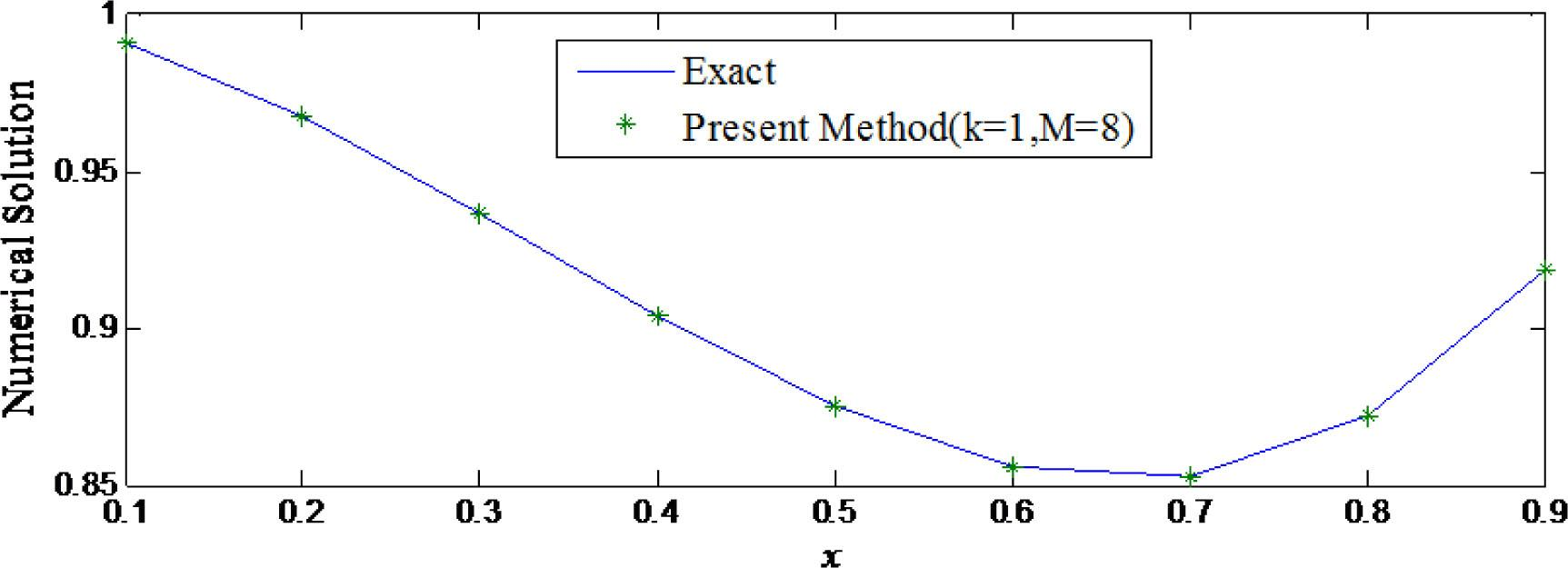Comparison of numerical solutions with exact solutions of example 1.

Numerical results of example 1.

xExact solutionPresent method (k = 1, M = 8)Abs. Error
0.10.99100.99101.61e-12
0.20.96800.96804.71e-13
0.30.93700.93702.27e-12
0.40.90400.90403.04e-12
0.50.87500.87502.52e-12
0.60.85600.85609.67e-13
0.70.85300.85309.64e-13
0.80.87200.87202.36e-12
0.90.91900.91902.36e-12

Example 2

Consider the Abel′s integral equation of the first kind [22, 23],

$x=∫0xy(t)x−tdt,0≤x≤1.$$$\begin{array}{} \displaystyle x= \int_{0}^{x}\dfrac{y(t)}{\sqrt{x-t}}dt, \, \, \, \, 0\le x\le 1. \end{array}$$

which has the exact solution $y(x)=2πx.$$\begin{array}{} \displaystyle y(x)=\dfrac{2}{\pi} \sqrt{x}. \end{array}$ We solved the eq.(14) using the present method and obtained approximate solution is compared with exact and other existing methods which reflects in table 2 and figure 2. Error analysis is shown in table 3 and figure 3.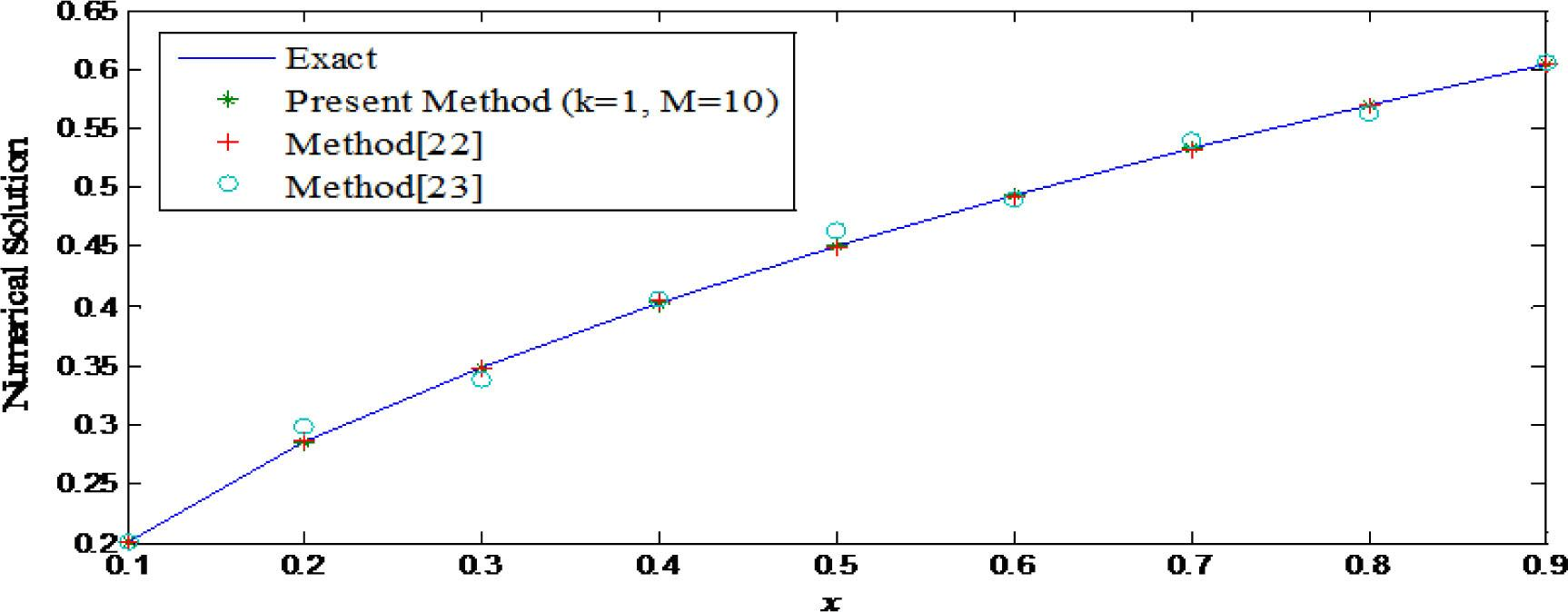Comparison of numerical solutions, exact solutions and existing methods of example 2.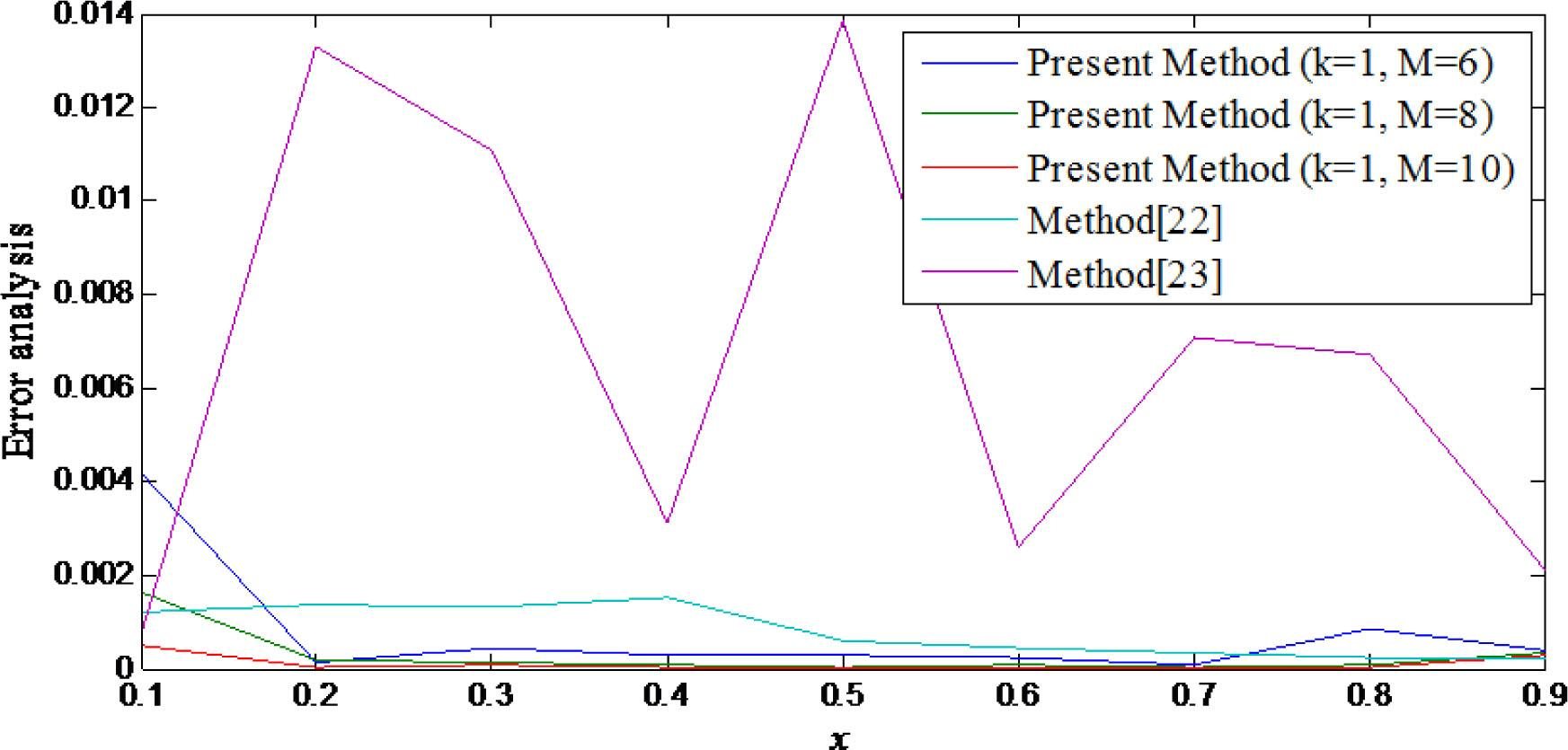Comparison of error analysis of example 2.

Numerical results of example 2.

xExact solutionPresent method (k = 1, M = 10)Method  (k = 1, M = 8)Method  (m = 16)
0.10.2013170.2008420.2001280.200460
0.20.2847050.2846670.2860920.297987
0.30.3486910.3486280.3473940.337588
0.40.4026340.4026090.4041610.405769
0.50.4501580.4501290.4495680.464014
0.60.4931240.4931130.4927040.490550
0.70.5326340.5326070.5323150.539721
0.80.5694100.5694400.5691560.562698
0.90.6039510.6036900.6037420.606044

Error analysis of example 2.

xPresent method (k = 1, M = 10)Method  (k = 1, M = 8)Method  (m = 16)
0.14.73e-041.18e-038.57e-04
0.23.77e-051.38e-031.32e-02
0.36.21e-051.29e-031.11e-02
0.42.37e-051.52e-033.13e-03
0.52.85e-055.90e-041.38e-02
0.69.87e-064.19e-042.57e-03
0.72.72e-053.19e-047.08e-03
0.83.05e-052.54e-046.71e-03
0.92.59e-042.08e-042.09e-03

Example 3

Consider the Abel′s integral equation of the second kind [22, 23],

$4y(x)=4x+1−arcsin1−x1+x+π2−∫0xy(t)x−tdt,0≤x≤1.$$$\begin{array}{} \displaystyle 4y(x)=\dfrac{4}{\sqrt{x+1}}- arcsin \left( \dfrac{1-x}{1+x} \right) + \dfrac{\pi}{2} - \int_{0}^{x} \dfrac{y(t)}{\sqrt{x-t}} dt, \, \, \, \, 0\le x\le 1. \end{array}$$

which has the exact solution $y(x)=1x+1.$$\begin{array}{} \displaystyle y(x)=\dfrac{1}{\sqrt{x+1}}. \end{array}$ Applying the Hermite wavelet method for solving eq.(15), then obtained approximate solution is compared with the exact solution and method are shown in table 4 and figure 4. Error analysis is shown in table 5.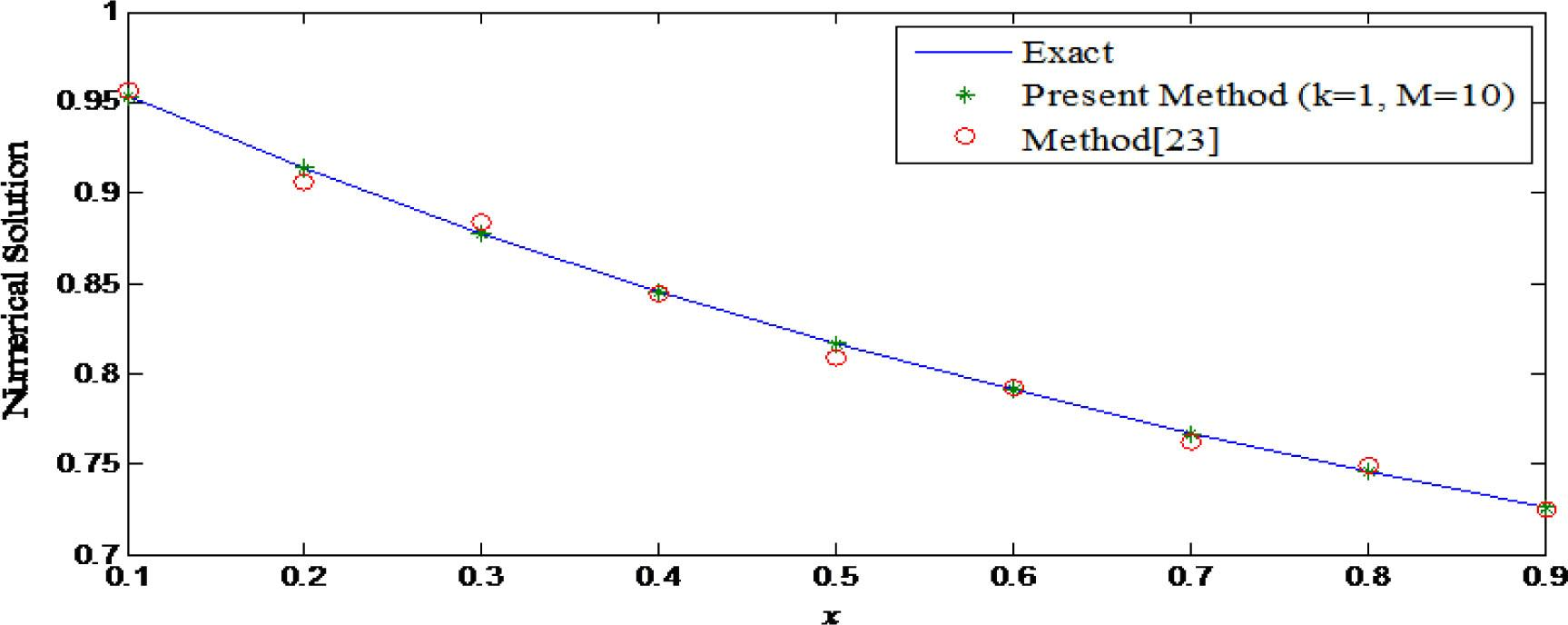Comparison of Numerical solutions of example 3.

Numerical results of example 3.

xExact solutionPresent method (k = 1, M = 10)Method  (m = 16)
0.10.9534625892455920.9534626044535200.95646081381695
0.20.9128709291752770.9128709284827000.90601007037324
0.30.8770580193070290.8770580211058820.88361513925322
0.40.8451542547285170.8451542553083540.84340093819493
0.50.8164965809277260.8164965819764080.80822420481499
0.60.7905694150420950.7905694154217440.79221049469412
0.70.7669649888473700.7669649899960250.76284677221990
0.80.7453559924999300.7453559915059690.74933888037055
0.90.7254762501100120.7254762589759920.72434536240934

Error analysis of example 3.

xPresent method (k = 1, M = 10)Method  (m = 16)
0.11.52e-082.99e-03
0.26.92e-106.86e-03
0.31.79e-096.55e-03
0.45.79e-101.75e-03
0.51.04e-098.27e-03
0.63.79e-101.64e-03
0.71.14e-094.11e-03
0.89.93e-103.98e-03
0.98.86e-091.13e-03

Example 4

Consider the Abel′s integral equations of the second kind [22, 23],

$y(x)=2x−∫0xy(t)x−tdt,0≤x≤1.$$$\begin{array}{} \displaystyle y(x)=2\sqrt{x}- \int_{0}^{x} \dfrac{y(t)}{\sqrt{x-t}}dt, \, \, \, \, 0\le x\le 1. \end{array}$$

which has the exact solution $y(x)=1−exp(πt)erfc(πt).$$\begin{array}{} \displaystyle y(x)=1-exp(\pi t) erfc{(\sqrt{\pi t)} }. \end{array}$ We solved the eq.(16) by the present method, we obtain the approximate solution and is compared with exact and other existing methods as shown in table 6 and figure 5. Error analysis is shown in table 7 and figure 6.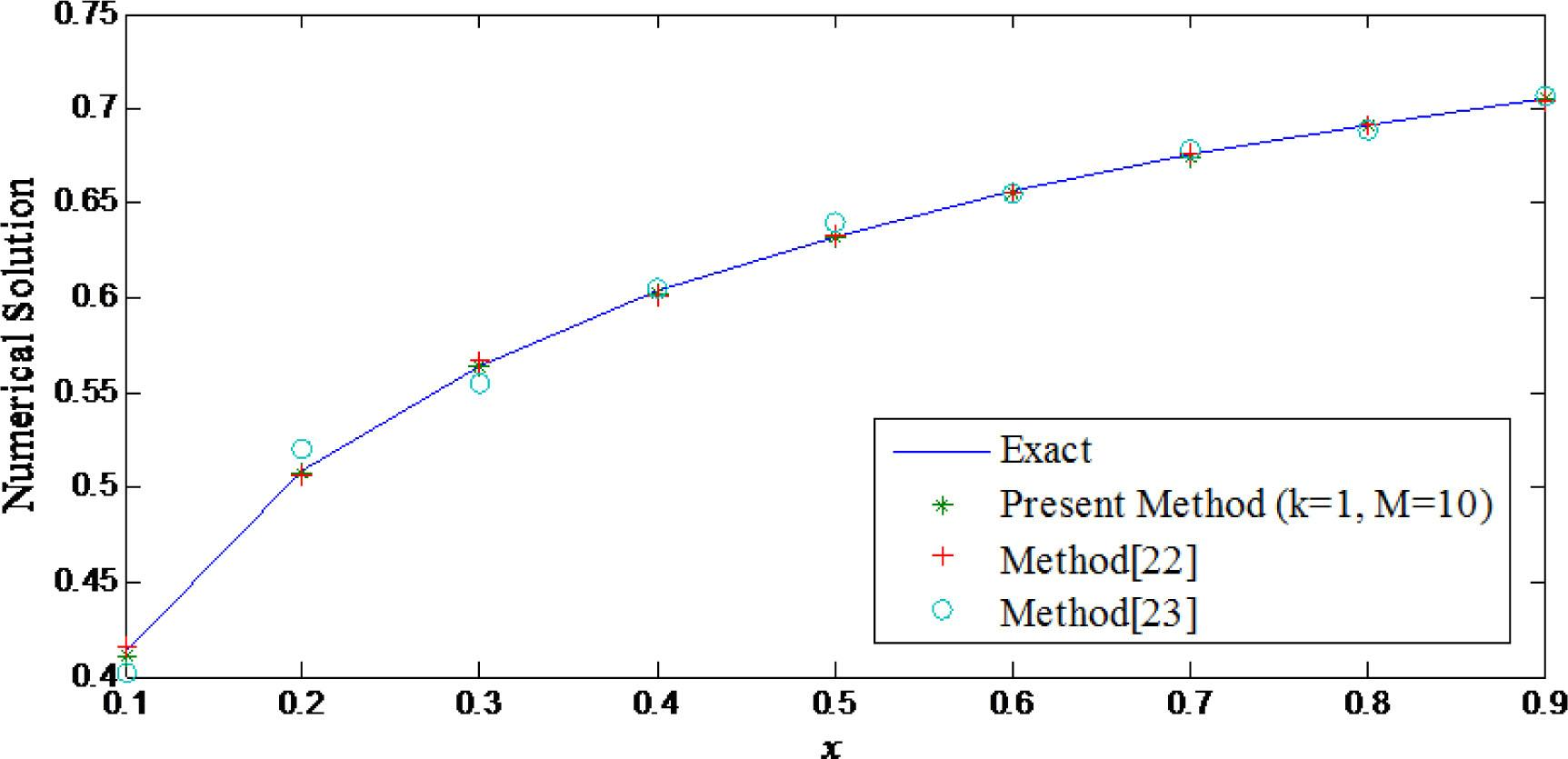Comparison of Numerical solutions of example 4.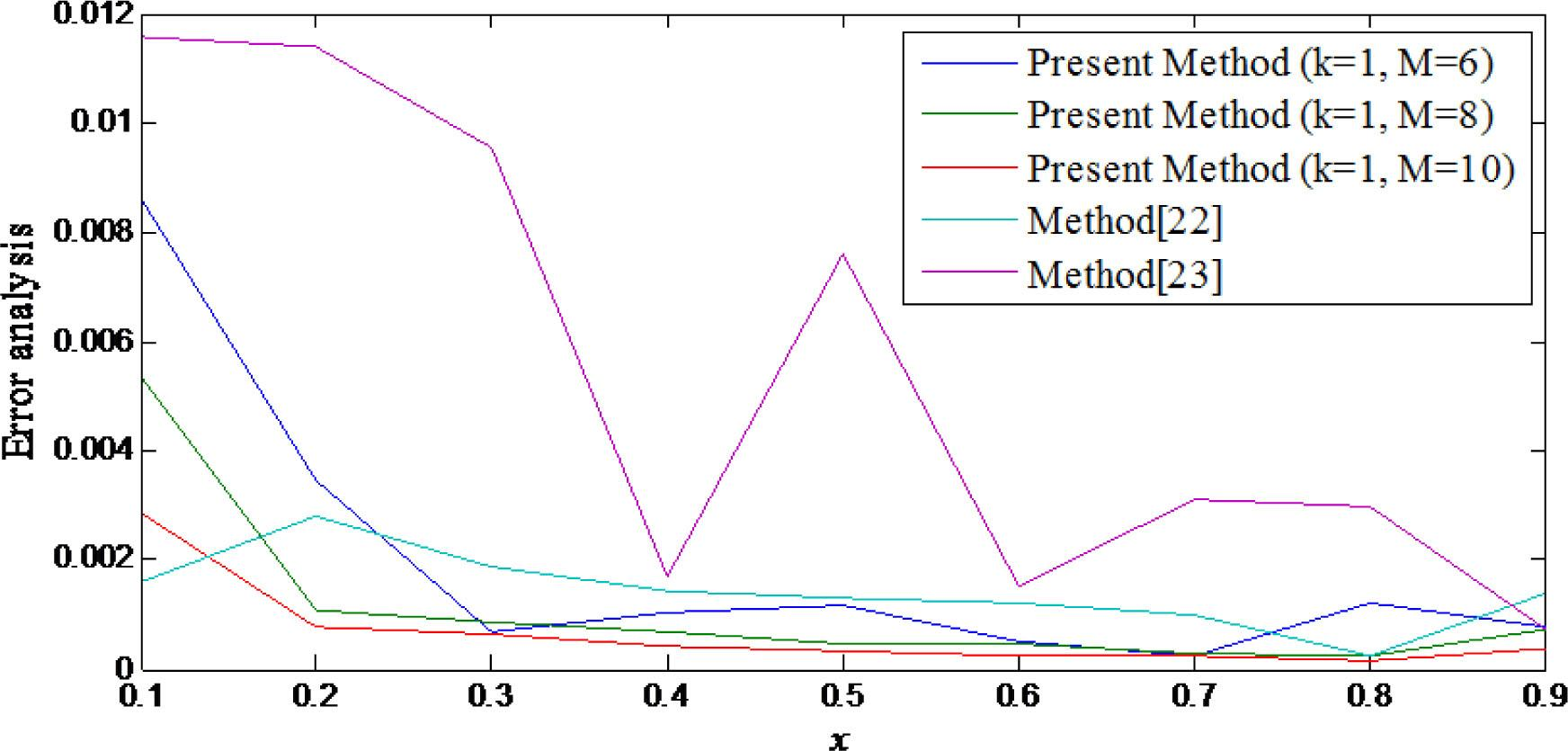Comparison of Error analysis of example 4.

Numerical results of example 4.

xExact solutionPresent method (k = 1, M = 10)Method  (k = 0, M = 16)Method  (m = 16)
0.10.4140590.4112290.4156890.402472
0.20.5083520.5075720.5055280.519751
0.30.5643090.5636850.5662050.554755
0.40.6033470.6029260.6019080.605031
0.50.6328680.6325210.6341880.640487
0.60.6563230.6560590.6551090.654785
0.70.6756010.6753580.6765880.678700
0.80.6918420.6916900.6915960.688860
0.90.7057870.7053980.7043770.706495

Error analysis of example 4.

xPresent method (k = 1, M = 10)Method  (k = 0, M = 16)Method  (m = 16)
0.12.82e-031.62e-031.15e-02
0.27.79e-042.82e-031.13e-02
0.36.23e-041.89e-039.55e-03
0.44.21e-041.43e-031.68e-03
0.53.46e-041.32e-037.61e-03
0.62.63e-041.21e-031.53e-03
0.72.42e-049.86e-043.09e-03
0.81.50e-042.45e-042.98e-03
0.93.87e-041.40e-037.08e-04

Conclusion

The Hermite wavelet method is applied for the numerical solution of Abel′s integral equations. The present method reduces an integral equation into a set of algebraic equations. Obtained results are higher accuracy with exact ones and existing methods [22, 23], which can be observed in section 5. The numerical results shows that the accuracy improves with increasing the values of M for better accuracy. Convergence theorem reveals that existence of solution.Comparison of numerical solutions with exact solutions of example 1.Comparison of numerical solutions, exact solutions and existing methods of example 2.Comparison of error analysis of example 2.Comparison of Numerical solutions of example 3.Comparison of Numerical solutions of example 4.Comparison of Error analysis of example 4.

#### Numerical results of example 4.

xExact solutionPresent method (k = 1, M = 10)Method  (k = 0, M = 16)Method  (m = 16)
0.10.4140590.4112290.4156890.402472
0.20.5083520.5075720.5055280.519751
0.30.5643090.5636850.5662050.554755
0.40.6033470.6029260.6019080.605031
0.50.6328680.6325210.6341880.640487
0.60.6563230.6560590.6551090.654785
0.70.6756010.6753580.6765880.678700
0.80.6918420.6916900.6915960.688860
0.90.7057870.7053980.7043770.706495

#### Error analysis of example 3.

xPresent method (k = 1, M = 10)Method  (m = 16)
0.11.52e-082.99e-03
0.26.92e-106.86e-03
0.31.79e-096.55e-03
0.45.79e-101.75e-03
0.51.04e-098.27e-03
0.63.79e-101.64e-03
0.71.14e-094.11e-03
0.89.93e-103.98e-03
0.98.86e-091.13e-03

#### Error analysis of example 2.

xPresent method (k = 1, M = 10)Method  (k = 1, M = 8)Method  (m = 16)
0.14.73e-041.18e-038.57e-04
0.23.77e-051.38e-031.32e-02
0.36.21e-051.29e-031.11e-02
0.42.37e-051.52e-033.13e-03
0.52.85e-055.90e-041.38e-02
0.69.87e-064.19e-042.57e-03
0.72.72e-053.19e-047.08e-03
0.83.05e-052.54e-046.71e-03
0.92.59e-042.08e-042.09e-03

#### Error analysis of example 4.

xPresent method (k = 1, M = 10)Method  (k = 0, M = 16)Method  (m = 16)
0.12.82e-031.62e-031.15e-02
0.27.79e-042.82e-031.13e-02
0.36.23e-041.89e-039.55e-03
0.44.21e-041.43e-031.68e-03
0.53.46e-041.32e-037.61e-03
0.62.63e-041.21e-031.53e-03
0.72.42e-049.86e-043.09e-03
0.81.50e-042.45e-042.98e-03
0.93.87e-041.40e-037.08e-04

#### Numerical results of example 1.

xExact solutionPresent method (k = 1, M = 8)Abs. Error
0.10.99100.99101.61e-12
0.20.96800.96804.71e-13
0.30.93700.93702.27e-12
0.40.90400.90403.04e-12
0.50.87500.87502.52e-12
0.60.85600.85609.67e-13
0.70.85300.85309.64e-13
0.80.87200.87202.36e-12
0.90.91900.91902.36e-12

#### Numerical results of example 3.

xExact solutionPresent method (k = 1, M = 10)Method  (m = 16)
0.10.9534625892455920.9534626044535200.95646081381695
0.20.9128709291752770.9128709284827000.90601007037324
0.30.8770580193070290.8770580211058820.88361513925322
0.40.8451542547285170.8451542553083540.84340093819493
0.50.8164965809277260.8164965819764080.80822420481499
0.60.7905694150420950.7905694154217440.79221049469412
0.70.7669649888473700.7669649899960250.76284677221990
0.80.7453559924999300.7453559915059690.74933888037055
0.90.7254762501100120.7254762589759920.72434536240934

#### Numerical results of example 2.

xExact solutionPresent method (k = 1, M = 10)Method  (k = 1, M = 8)Method  (m = 16)
0.10.2013170.2008420.2001280.200460
0.20.2847050.2846670.2860920.297987
0.30.3486910.3486280.3473940.337588
0.40.4026340.4026090.4041610.405769
0.50.4501580.4501290.4495680.464014
0.60.4931240.4931130.4927040.490550
0.70.5326340.5326070.5323150.539721
0.80.5694100.5694400.5691560.562698
0.90.6039510.6036900.6037420.606044

C. K. Chui, Wavelets: A Mathematical Tool for Signal Analysis, SIAM, Philadelphia, PA., 1997.ChuiC. K.Wavelets: A Mathematical Tool for Signal AnalysisSIAMPhiladelphia, PA1997Search in Google Scholar

G. Beylkin, R. Coifman, V. Rokhlin, Fast wavelet transforms and numerical algorithms I, Commun. Pure Appl. Math., 44 (1991), 141–183.BeylkinG.CoifmanR.RokhlinV.Fast wavelet transforms and numerical algorithms ICommun. Pure Appl. Math.441991141183Search in Google Scholar

Ü. Lepik, E. Tamme, Application of the Haar wavelets for solution of linear integral Equations, Ant. Turk–Dynam. Sys. Appl. Proce., (2005), 395–407.LepikÜ.TammeE.Application of the Haar wavelets for solution of linear integral EquationsAnt. Turk–Dynam. Sys. Appl. Proce.2005395407Search in Google Scholar

K. Maleknejad, M.T. Kajani, Y. Mahmoudi, Numerical solution of linear Fredholm and Volterra integral equation of the second kind by using Legendre wavelets, J. Kybernet., 32 (2003), 1530-1539.MaleknejadK.KajaniM.T.MahmoudiY.Numerical solution of linear Fredholm and Volterra integral equation of the second kind by using Legendre waveletsJ. Kybernet.32200315301539Search in Google Scholar

K. Maleknejad, F. Mirzaee, Using rationalized haar wavelet for solving linear integral equations, App. Math. Comp., 160 (2005), 579–587.MaleknejadK.MirzaeeF.Using rationalized haar wavelet for solving linear integral equationsApp. Math. Comp.1602005579587Search in Google Scholar

K. Maleknejad, M. Yousefi, Numerical solution of the integral equation of the second kind by using wavelet bases of hermite cubic splines, App. Math. Comp., 183 (2006), 134-141.MaleknejadK.YousefiM.Numerical solution of the integral equation of the second kind by using wavelet bases of hermite cubic splinesApp. Math. Comp.1832006134141Search in Google Scholar

K. Maleknejad, T. Lotfi, Y. Rostami, Numerical computational method in solving fredholm integral equations of the second kind by using coifman wavelet, App. Math. Comp., 186 (2007), 212-218.MaleknejadK.LotfiT.RostamiY.Numerical computational method in solving fredholm integral equations of the second kind by using coifman waveletApp. Math. Comp.1862007212218Search in Google Scholar

S. Yousefi, A. Banifatemi, Numerical solution of Fredholm integral equations by using CAS wavelets, App. Math. Comp., 183 (2006), 458-463.YousefiS.BanifatemiA.Numerical solution of Fredholm integral equations by using CAS waveletsApp. Math. Comp.1832006458463Search in Google Scholar

S. C. Shiralashetti, R. A. Mundewadi, Bernoulli Wavelet Based Numerical Method for Solving Fredholm Integral Equations of the Second Kind, J. Inform. Comp. Sci., 11(2) (2016), 111-119.ShiralashettiS. C.MundewadiR. A.Bernoulli Wavelet Based Numerical Method for Solving Fredholm Integral Equations of the Second KindJ. Inform. Comp. Sci.1122016111119Search in Google Scholar

S. A. Yousefi, Numerical solution of Abel’s integral equation by using Legendre wavelets, Appl. Math. Comp., 175 (2006), 574–80.YousefiS. A.Numerical solution of Abel’s integral equation by using Legendre waveletsAppl. Math. Comp.175200657480Search in Google Scholar

S. Sohrabi, Comparison Chebyshev wavelets method with BPFs method for solving Abel’s integral equation, Ain Shams Eng. J., 2 (2011), 249–254.SohrabiS.Comparison Chebyshev wavelets method with BPFs method for solving Abel’s integral equationAin Shams Eng. J.22011249254Search in Google Scholar

A. M. Wazwaz, Linear and nonlinear integral equations: methods and applications, Berlin: Higher Education, Beijing, Springer, 2011.WazwazA. M.Linear and nonlinear integral equations: methods and applicationsBerlin: Higher Education, BeijingSpringer2011Search in Google Scholar

A. M. Wazwaz, A first course in integral equations, Singapore: World Scientific Publishing, 1997.WazwazA. M.A first course in integral equationsSingaporeWorld Scientific Publishing1997Search in Google Scholar

R. Gorenflo, S. Vessella, Abel integral equations, analysis and applications, In: Lecture notes in mathematics, Heidelberg: Springer, 1991.GorenfloR.VessellaS.Abel integral equations, analysis and applicationsLecture notes in mathematicsHeidelbergSpringer1991Search in Google Scholar

C.T.H. Baker, The numerical treatment of integral equations, Clarendon Press, Oxford, 1977.BakerC.T.H.The numerical treatment of integral equationsClarendon PressOxford1977Search in Google Scholar

S.C. Shiralashetti, S. Kumbinarasaiah, Theoretical study on continuous polynomial wavelet bases through wavelet series collocation method for nonlinear Lane–Emden type equations, Appl. Math. Comput., 315 (2017), 591–602.ShiralashettiS.C.KumbinarasaiahS.Theoretical study on continuous polynomial wavelet bases through wavelet series collocation method for nonlinear Lane–Emden type equationsAppl. Math. Comput.3152017591602Search in Google Scholar

H. Brunner, Collocation methods for Volterra integral and related functional differential equations, Cambridge Monographs on Applied and Computational Mathematics, Cambridge Univ. Press, Cambridge, 2004.BrunnerH.Collocation methods for Volterra integral and related functional differential equationsCambridge Monographs on Applied and Computational MathematicsCambridge Univ. PressCambridge2004Search in Google Scholar

S. Noeiaghdam, E. Zarei, H. B. Kelishami, Homotopy analysis transform method for solving Abel’s integral equations of the first kind, Ain Shams Eng. J., 7 (2016), 483–495.NoeiaghdamS.ZareiE.KelishamiH. B.Homotopy analysis transform method for solving Abel’s integral equations of the first kindAin Shams Eng. J.72016483495Search in Google Scholar

N. Zeilon, Sur quelques points de la theorie de l’equation integrale d’Abel, Arkiv Mat Astr Fysik, 18 (1924), 1–19.ZeilonN.Sur quelques points de la theorie de l’equation integrale d’AbelArkiv Mat Astr Fysik181924119Search in Google Scholar

C.T.H. Baker, The numerical treatment of integral equations, Oxford: Clarendon Press, 1977.BakerC.T.H.The numerical treatment of integral equationsOxfordClarendon Press1977Search in Google Scholar

P. Baratella, A. P. Orsi, A new approach to the numerical solution of weakly singular Volterra integral equations, J. Comput. Appl. Math., 163(2) (2004), 401–418.BaratellaP.OrsiA. P.A new approach to the numerical solution of weakly singular Volterra integral equationsJ. Comput. Appl. Math.16322004401418Search in Google Scholar

 E. Babolian, A. Salimi Shamloo, Numerical solution of Volterra integral and integro-differential equations of convolution type by using operational matrices of piecewise constant orthogonal functions, J. Comp. Appl. Math., 214 (2008), 495–508.BabolianE.SalimiShamloo A.Numerical solution of Volterra integral and integro-differential equations of convolution type by using operational matrices of piecewise constant orthogonal functionsJ. Comp. Appl. Math.2142008495508Search in Google Scholar

A. Shahsavaran, M. R. Moazami Goudarzi, O. Moradtalab, Solving Abel’s integral equation of the first kind using piecewise constant functions and Taylor expansion by collocation method, In: 40th Annual Iranian mathematics conference.ShahsavaranA.MoazamiGoudarzi M. R.MoradtalabO.Solving Abel’s integral equation of the first kind using piecewise constant functions and Taylor expansion by collocation method40th Annual Iranian mathematics conferenceSearch in Google Scholar

A. Ali, M.A. Iqbal, S.T. Mohyud-Din, Hermites wavelets method for Boundary Value problems, Inter. J. Modern Appl. Phy., 3(1) (2013), 38-47.AliA.IqbalM.A.Mohyud-DinS.T.Hermites wavelets method for Boundary Value problemsInter. J. Modern Appl. Phy.3120133847Search in Google Scholar

M.H. Kantli, S.C. Shiralashetti, Finite difference Wavelet–Galerkin method for the numerical solution of elastohydrodynamic lubrication problems, Journal of Analysis, 26(2) (2018), 285-295.KantliM.H.ShiralashettiS.C.Finite difference Wavelet–Galerkin method for the numerical solution of elastohydrodynamic lubrication problemsJournal of Analysis2622018285295Search in Google Scholar

N.M. Bujurke, M.H. Kantli, B.M. Shettar, Jacobian free Newton-GMRES method for the solution of elastohydrodynamic grease lubrication in line contact using wavelet based pre-conditioners, Proceedings of the National Academy of Sciences, India Section A: Physical Sciences, 88(2) (2018), 247-265.BujurkeN.M.KantliM.H.ShettarB.M.Jacobian free Newton-GMRES method for the solution of elastohydrodynamic grease lubrication in line contact using wavelet based pre-conditioners, Proceedings of the National Academy of SciencesIndia Section A: Physical Sciences8822018247265Search in Google Scholar

N.M. Bujurke, M. H. Kantli, B.M. Shettar, Wavelet preconditioned Newton-Krylov method for elastohydrodynamic lubrication of line contact problems, Applied Mathematical Modelling, 46 (2017), 285-298.BujurkeN.M.KantliM. H.ShettarB.M.Wavelet preconditioned Newton-Krylov method for elastohydrodynamic lubrication of line contact problemsApplied Mathematical Modelling462017285298Search in Google Scholar

S.C. Shiralashetti, M.H. Kantli, A.B. Deshi, A new wavelet multigrid method for the numerical solution of elliptic type differential equations, Alexandria Engineering Journal, 57 (2018), 203-209.ShiralashettiS.C.KantliM.H.DeshiA.B.A new wavelet multigrid method for the numerical solution of elliptic type differential equationsAlexandria Engineering Journal572018203209Search in Google Scholar